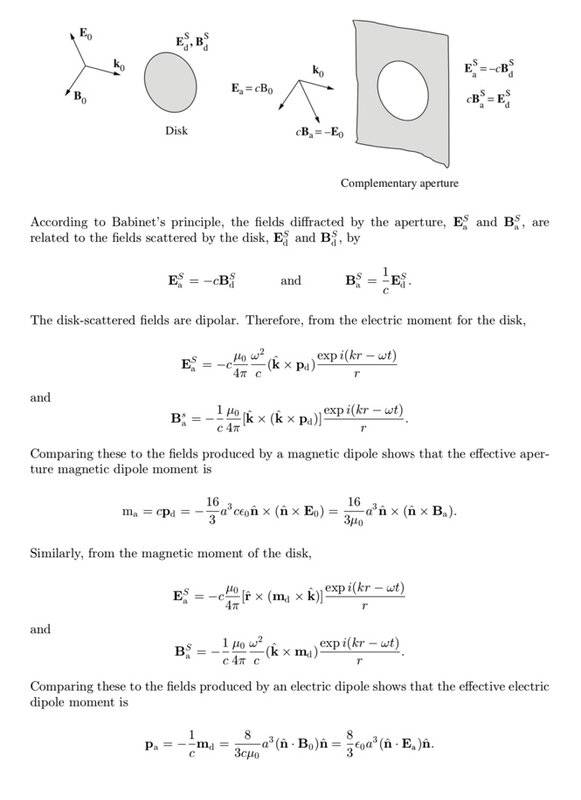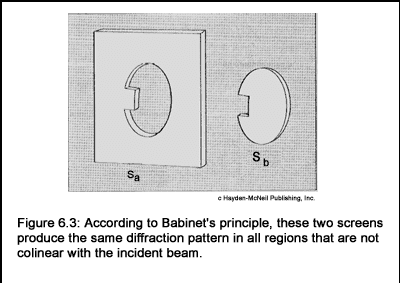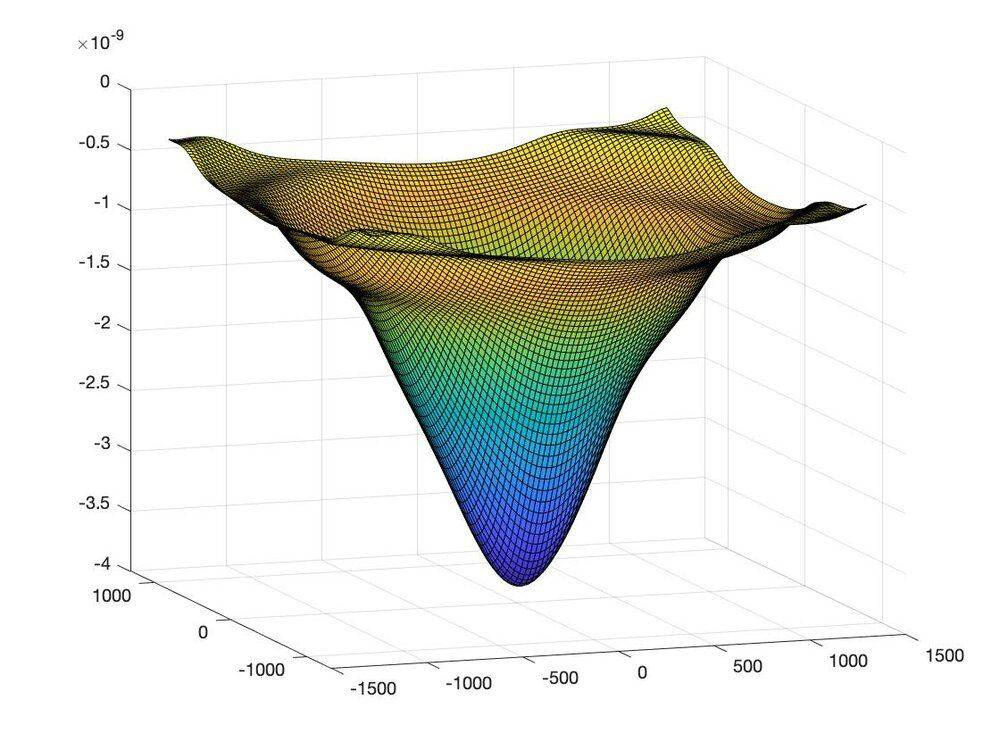# Babinet's Principle: Effective Aperture Dipoles (Matlab)

Homework Statement:
A monochromatic plane wave with fields ##\vec{E}_0## and ##\vec{B}_0## scatters from a thin conducting disk of radius ##a##. In the long-wavelength limit, the scattered field is described by electric and magnetic dipole radiation fields with moments

##\vec{p}_d = - \frac{16}{3} a^3 \epsilon_0 \hat{n} \times \left( \hat{n} \times \vec{E}_0 \right)##

and

##\vec{m}_d = - \frac{8}{3 \mu_0} a^3 \left( \hat{n} \cdot \vec{B}_0 \right) \hat{n}##

The unit vector ##\hat{n}## points in the direction of the incident wave propagation vector when the latter is normal to the plane of the disk. Use Babinet's principle to deduce the effective dipole moments which characterize the diffracted field when a circular hole of radius ##a## in a flat conducting plane is illuminated by a plane wave with aperture fields ##\vec{E}_a## and ##\vec{B}_a##.

I want to program a diffraction flux pattern from a circular aperture in an infinite plane perfect conductor. I think the result I'm trying to get is a Bessel function type profile with a central maxima. I think this is valid when assuming normal incidence which is what I'll assume from here on out. Maybe I misunderstand Babinet's principle and could use some help/clarification.
Relevant Equations:
See solution below.For clarification on "normal incidence" without drawing a picture.

I'm going to assume the incoming wave has the electric field in the z-direction, the magnetic B / Auxillary H-field in the x-direction, and the Poynting Vector in the y-direction (i.e. normal to the plane of the aperture).

That being the case,

##\vec{m}_a = \frac{16}{3} a^3 \epsilon_0 \vec{E}_0 \left( \hat{n} \cdot \hat{n} \right) = \frac{16}{3} z^3 \epsilon_0 E_0 \hat{y} ##

and

##\vec{p}_a = 0##

So now we have to calculate the fields from effective aperture magnetic dipole, we can do this directly from the magnetic vector potential which according to Jackson 9.33 is

##\vec{A} \left( \vec{r} \right) = \frac{i k \mu_0}{4 \pi} \left( \hat{r} \times \vec{m} \right) \frac{e^{ikr}}{r} \left( 1 - \frac{1}{ikr} \right)##

for a time harmonic oscillating source

Of course

##\vec{H} = \frac{1}{\mu} \nabla \times \vec{A}##

I think

##\vec{E} = \frac{i}{k}\sqrt{\frac{\mu}{\epsilon}} \nabla \times \vec{H}##

Both of which can be numerically computed

When I add back the plane waves per Babinet's principle I get the following

which is nothing like the typical Bessel function like diffraction profile.

Can someone walk me through what I should be doing, and how I should be interpreting it?

It should be worth noting that I found the total fields E and H before finding the total poynting vector so I did not neglect cross terms

#### Attachments

•etotheipiI think I’m going to try and solve the analogous scattering problem of a circular disk with normal incidence and see what I can learn from it. Hopefully, I actually have enough knowledge of the relevant PDE solutions and basis functions. I’m not the most knowledgeable when it comes to Bessel functions.Several things

1) My flux pattern is upside down

2) It looks like it could be cylindrically symmetric but it's not dipping to zero and coming back up (or down rather) even when I extend my views. There shouldn't be shelves of sorts.

3) For my plane wave in the y-direction I arbitrarily made it ##E_z = E_0 \frac{e^{iky}}{r}## (polarized in z-direction). That inverse r power should not be there, it's extremely contrived but it has worked better than anything I've done thus far.